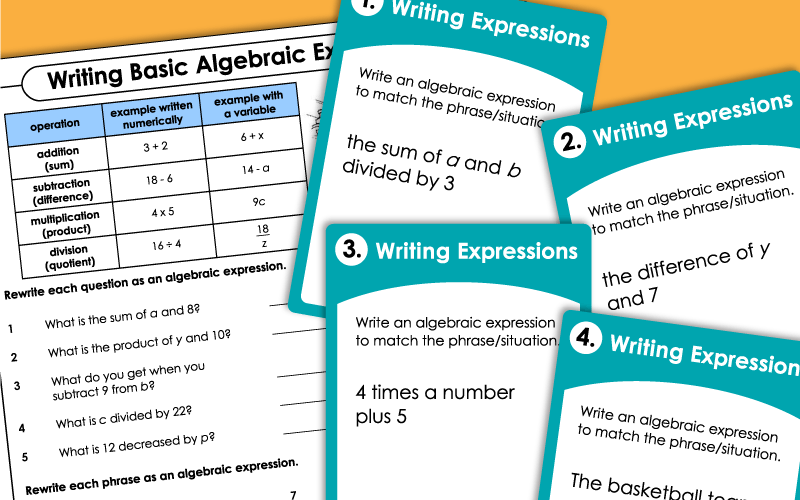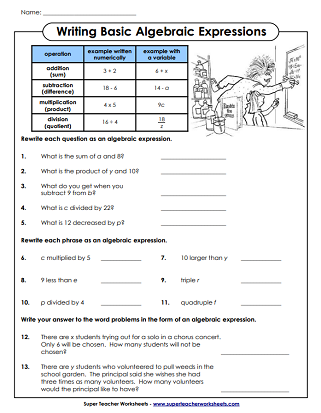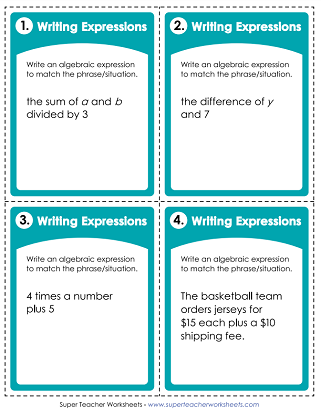# Writing Algebraic Expressions

Practice writing expressions with variables with these printable worksheets and task cards.## Level: Basic(1-Step; No Parenthesis)

Write algebraic expressions. example: What is the sum of a and 8? - a+8
Match the one-step algebraic expressions with the situations described in words.
Use these 30 task cards to help your students learn to write algebraic expressions. These cards work well for classroom scavenger hunts, peer tutoring, learning centers, and document cameras.

On this worksheet, students will use variables to write algebraic expressions to represent the 10 situations described.
On this worksheet, students write two-step expressions. Many include variables.
Print out this worksheet and have your students match the two-step algebraic expressions on the left to the correct situations on the right.
Write an expression for each situation given. This set includes 30 task cards.
More Algebra Topics

We have lots of pre-algebra and algebra worksheets on a variety of topics. Worksheets include: evaluating expressions, solving one-step equations, solving two-step equations, dependent and independent variables, and inequalities.

Evaluating Expressions

## Sample Worksheet ImagesMy Account
Site Information